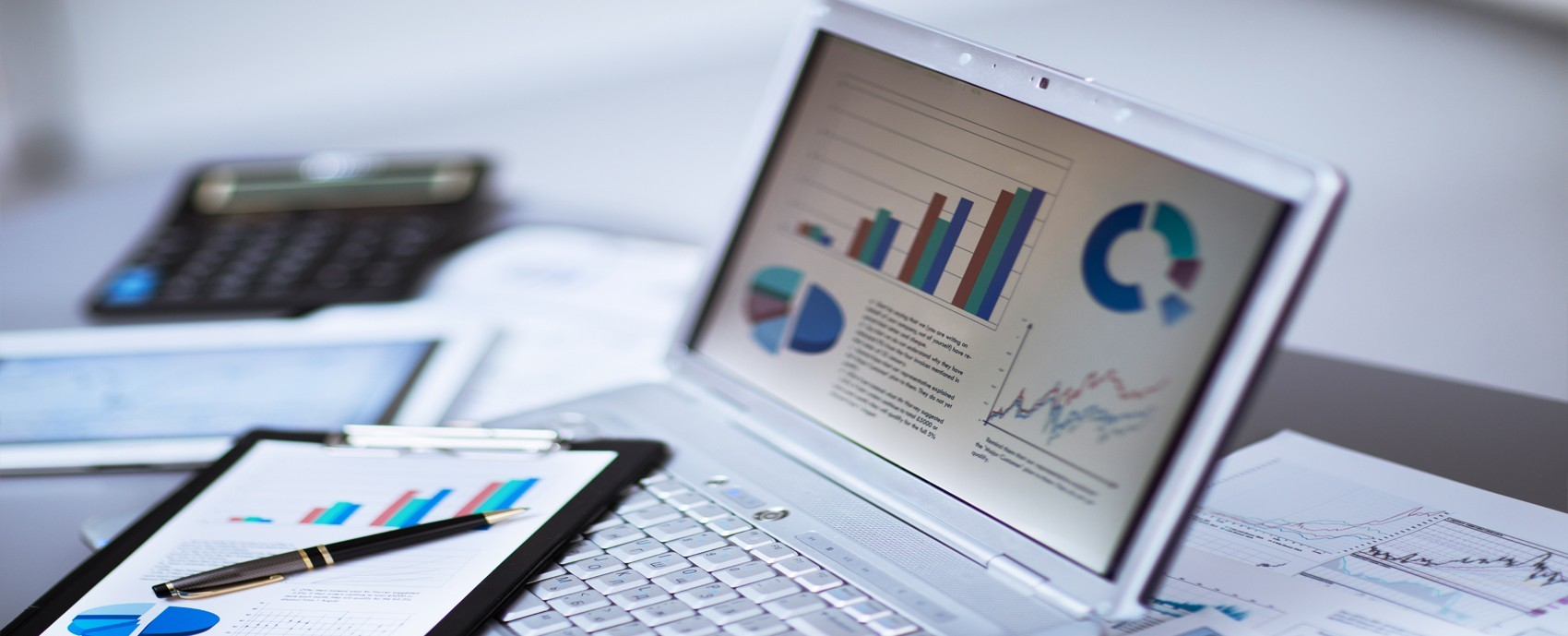# Cost Accounting – Classification of Cost

## Accounting Softwares

Costs can be classified based on the following attributes:

## By Nature

In this type, material, labor and overheads are three costs, which can be further sub-divided into raw materials, consumables, packing materials, and spare parts etc.

## By Degree of Traceability of the Product

Direct and indirect expenses are main types of costs come under it. Direct expenses may directly attributable to a particular product. Leather in shoe manufacturing is a direct expenses and salaries, rent of building etc. come under indirect expenses in the accounting softwares.

## By Controllability

In this classification, two types of costs fall:

• Controllable – These are controlled by management like material labour and direct expenses.
• Uncontrollable – They are not influenced by management or any group of people. They include rent of a building, salaries, and other indirect expenses.

## By Relationship with Accounting Period

Classifications are measured by the period of use and benefit. The capital expenditure and revenue expenditure are classified under it. Revenue expenses relate to current accounting period. Capital expenditures are the benefits beyond accounting period. Fixed assets come under category of capital expenditure and maintenance of assets comes under revenue expenditure category.

## By Association with the Product

There are two categories under this classification:

• Product cost – Product cost is identifiable in any product. It includes direct material, direct labor and direct overheads. Up to sale, these products are shown and valued as inventory and they form a part of balance sheet. Any profitability is reflected only when these products are sold. The Costs of these products are transferred to costs of goods sold account.
• Time/Period base cost – Selling expenditure and Administrative expenditure, both are time or period based expenditures. For example, rent of a building, salaries to employees are related to period only. Profitability and costs are depends on both, product cost and time/period cost.

## By Functions

Under this category, the cost is divided by its function as follows:

• Production Cost – It represents the total manufacturing or production cost.
• Commercial cost – It includes operational expenses of the business and may be sub-divided into administration cost, and selling and distribution cost.

## By Change in Activity or Volume

Under this category, the cost is divided as fixed, variable, and semi-variable costs:

• Fixed cost – It mainly relates to time or period. It remains unchanged irrespective of volume of production like factory rent, insurance, etc. The cost per unit fluctuates according to the production. The cost per unit decreases if production increases and cost per unit increases if the production decreases. That is, the cost per unit is inversely proportional to the production. For example, if the factory rent is Rs 25,000 per month and the number of units produced in that month is 25,000, then the cost of rent per unit will be Rs 1 per unit. In case the production increases to 50,000 units, then the cost of rent per unit will be Rs 0.50 per unit.
• Variable cost – Variable cost directly associates with unit. It increases or decreases according to the volume of production. Direct material and direct labor are the most common examples of variable cost. It means the variable cost per unit remains constant irrespective of production of units.
• Semi-variable cost – A specific portion of these costs remains fixed and the balance portion is variable, depending on their use. For example, if the minimum electricity bill per month is Rs 5,000 for 1000 units and excess consumption, if any, is charged @ Rs 7.50 per unit. In this case, fixed electricity cost is Rs 5,000 and the total cost depends on the consumption of units in excess of 1000 units. Therefore, the cost per unit up to a certain level changes according to the volume of production, and after that, the cost per unit remains constant @ Rs 7.50 per unit.

SOURCE: TUTORIALSPOINT.COM